# Precalculus : Sequences and Series

## Example Questions

1 2 3 5 Next →

### Example Question #6 : Sums Of Infinite Series

Find the sum of the following infinite series: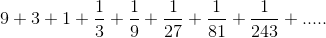Possible Answers: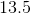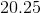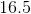Correct answer:Explanation:

Notice that this is an infinite geometric series, with ratio of terms = 1/3. Hence it can be rewritten as: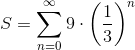Since the ratio, 1/3, has absolute value less than 1, we can find the sum using this formula: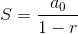Where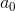is the first term of the sequence. In this case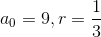, and thus: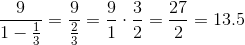### Example Question #7 : Sums Of Infinite Series

In the infinite series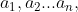each term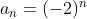such that the first two terms are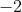and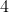.  What is the sum of the first eight terms in the series?

Possible Answers:

128

-256

210

-64

170

Correct answer:

170

Explanation:

Once you're identified the pattern in the series, you might see a quick way to perform the summation. Since the base of the exponent for each term is negative, the result will be positive if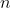is even, and negative if it is odd. And the series will just list the first 8 powers of 2, with that positive/negative rule attached. So you have:

-2, 4, -8, 16, -32, 64, -128, 256

Note that each "pair" of adjacent numbers has one negative and one positive. for the first pair, -2 + 4 = 2.  For the second, -8 + 16 = 8.  For the third, -32 + 64 = 32. And so for the fourth, -128 + 256 = 128.  You can then quickly sum the values to see that the answer is 170.

1 2 3 5 Next →

### All Precalculus Resources